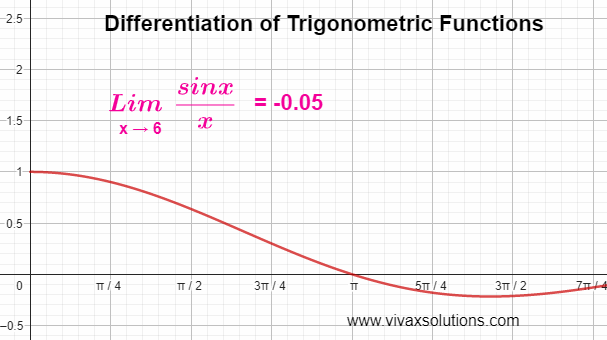## Posts

Showing posts from December 11, 2022

### Differentiating Trigonometric FunctionsWhen it comes to differentiating the functions of sin x and cos x, we need to use two fractions of the functions in question, when the variable approaches zero must be considered. They are as follows: lim sin (x) / x, as x → 0 lim (cos x - 1) / x as x → 0 Based on the above, sin x and cos x can be differentiated as follows: y = sin x → dy/dx = cos x y = cos x → dy/dx = - sin x y = tan x  → dy/dx = sec² x Differentiation of sine function